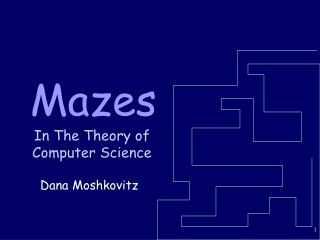# Mazes - PowerPoint PPT PresentationDownload PresentationMazes

MazesDownload Presentation## Mazes

- - - - - - - - - - - - - - - - - - - - - - - - - - - E N D - - - - - - - - - - - - - - - - - - - - - - - - - - -
##### Presentation Transcript

1. Mazes In The Theory of Computer Science Dana Moshkovitz

2. Can You Solve This Maze?

3. The Solution

4. Introduction • Objectives: • To explore the undirected connectivity problem • To introduce randomized computations • Overview: • Undirected Connectivity • Random Walks

5. Undirected Connectivity • Instance: An undirected graph G=(V,E) and two vertices s,tV • Problem: To decide if there is a path from s to t in G

6. What Do We Know? Theorem: Directed Connectivity is NL-Complete Corollary: Undirected Connectivity is in NL.

8. What If We Don’t Have “Magic Coins”? • Non-deterministic “algorithms” use “magic coins” to lead them to the right solution if one exists. • In real life, these are unavailable…

9. Idea! • What if we have plain coins? • In other words, what if we randomly choose a neighbor?

10. s t Random Walks Add a self loop to each vertex. • Start at s. • Let di be the degree of the current node. • Jump to each of the neighbors with probability 1/di. • Stop if you get to t.

11. s a t Notations • Let vt denote the node visited at time t (v0=s). • Let pt(i) = Pr[vt=i] p1(a)=0.5 p0(s)=1

12. Stationary Distribution Lemma: If G=(V,E) is a connected graph, for any iV,

13. Weaker Claim We’ll prove a weaker result: Lemma: If for some t, for any iV, then for any iV,

14. Proof Proof:di=2|E|. If the ith node has weight diat time t, then it retains this weight at time t+1 (it’s reachable (only) from its di neighbors). 

15. Illustrated Proof

16. Using the Asymptotic Estimate Corollary: Starting from some node i, we will revisit i within expectedly 2|E|/di steps.

17. One-Sided Error • Note that if the right answer is ‘NO’, we clearly answer ‘NO’. • Thus, a random walk algorithm has one-sided error. • Such algorithms are called “Monte-Carlo” algorithms.

18. s t . . . How Many Steps Are Needed? If the right answer is ‘YES’, in how many steps do we expect to discover that? But every time we get here, we get a second chance! The probability we head in the right direction is 1/ds

19. How Many Steps Are Needed? • Since expectedly we return to each vertex after 2|E|/di steps, • We expect to head in the right direction after |E| steps (w.p. ½). • By the linearity of the expectation, we expect to encounter t in d(s,t)|E||V||E| steps.

20. Randomized Algorithm for Undirected Connectivity • Run the random walk from s for 2|V||E| steps. • If node t is ever visited, answer “there is a path from s to t”. • Otherwise, reply “there is probably no path from s to t”.

21. PAP 401-404 Main Theorem Theorem: The above algorithm • uses logarithmic space • always right for ‘NO’ instances. • errs with probability at most ½ for ‘YES’ instances. To maintain the current position we only need log|V| space Markov: Pr(X>2E[X])<½

22. Summary • We explored the undirected connectivity problem. • We saw a log-space randomized algorithm for this problem. • We used an important technique called random walks.